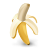# Counter

How many objects are in the figure below?A. $12$
B. $9$
C. $13$
D. $14$
E. $17$